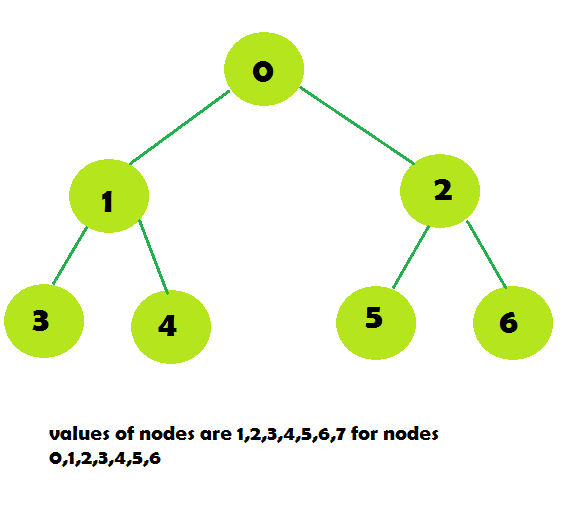# XOR of all the nodes in the sub-tree of the given node

Given an n-ary tree and Q queries where each query consists of an integer u which denotes a node. The task is to print the xor of all the values of nodes in the sub-tree of the given node.

Examples:

Input:q[] = {0, 1, 4, 5}
Output:
0
3
5
6
Query 1: (1 ^ 2 ^ 3 ^ 4 ^ 5 ^ 6 ^ 7) = 0
Query 2: (2 ^ 4 ^ 5) = 3
Query 3: (5) = 5
Query 4: (6) = 6

## Recommended: Please try your approach on {IDE} first, before moving on to the solution.

Naive approach: For each node we have to traverse the tree for every query find the xor of all the nodes of the sub-tree. So the complexity of the code will be O(N * Q).

Efficient approach: If we pre-compute the xor of all the nodes of the sub-tree by traversing the total tree once and first computing the xor of all the nodes of the sub-tree of the child node first and then using the value of the child node for computing the xor of all the nodes of the sub-tree of the parent node. In this way we can compute the xor in O(N) time and store them for queries. When a query is asked we will print the pre-computed value in O(1) time.

Below is the implementation of the above approach:

 `// C++ implementation of the approach ` `#include ` `using` `namespace` `std; ` ` `  `// Adjacency list of the graph ` `vector > graph; ` ` `  `// Value of the node ` `vector<``int``> values, xor_values; ` ` `  `// Function to pre-compute the xor values ` `int` `pre_compute_xor(``int` `i, ``int` `prev) ` `{ ` `    ``// xor of the sub-tree ` `    ``int` `x = values[i]; ` ` `  `    ``for` `(``int` `j = 0; j < graph[i].size(); j++) ` `        ``if` `(graph[i][j] != prev) { ` ` `  `            ``// xor x with xor of the sub-tree ` `            ``// of it child nodes ` `            ``x ^= pre_compute_xor(graph[i][j], i); ` `        ``} ` ` `  `    ``xor_values[i] = x; ` ` `  `    ``// Return the xor ` `    ``return` `x; ` `} ` ` `  `// Function to return the xor of ` `// the nodes of the sub-tree ` `// rooted at node u ` `int` `query(``int` `u) ` `{ ` `    ``return` `xor_values[u]; ` `} ` ` `  `// Driver code ` `int` `main() ` `{ ` `    ``int` `n = 7; ` ` `  `    ``graph.resize(n); ` `    ``xor_values.resize(n); ` ` `  `    ``// Create the graph ` `    ``graph.push_back(1); ` `    ``graph.push_back(2); ` `    ``graph.push_back(3); ` `    ``graph.push_back(4); ` `    ``graph.push_back(5); ` `    ``graph.push_back(6); ` ` `  `    ``// Set the values of the nodes ` `    ``values.push_back(1); ` `    ``values.push_back(2); ` `    ``values.push_back(3); ` `    ``values.push_back(4); ` `    ``values.push_back(5); ` `    ``values.push_back(6); ` `    ``values.push_back(7); ` ` `  `    ``// Pre-computation ` `    ``pre_compute_xor(0, -1); ` ` `  `    ``// Perform queries ` `    ``int` `queries[] = { 0, 1, 4, 5 }; ` `    ``int` `q = ``sizeof``(queries) / ``sizeof``(queries); ` `    ``for` `(``int` `i = 0; i < q; i++) ` `        ``cout << query(queries[i]) << endl; ` ` `  `    ``return` `0; ` `} `

Output:

```0
3
5
6
```

My Personal Notes arrow_drop_upSecond year Department of Information Technology Jadavpur University

If you like GeeksforGeeks and would like to contribute, you can also write an article using contribute.geeksforgeeks.org or mail your article to contribute@geeksforgeeks.org. See your article appearing on the GeeksforGeeks main page and help other Geeks.

Please Improve this article if you find anything incorrect by clicking on the "Improve Article" button below.

Article Tags :
Practice Tags :

Be the First to upvote.

Please write to us at contribute@geeksforgeeks.org to report any issue with the above content.